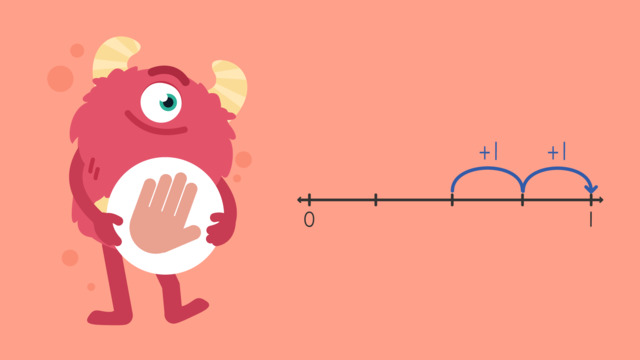# Adding Fractions on a Number Line — Let's Practice!Rating

Ø 5.0 / 1 ratings
The authorsTeam Digital
Adding Fractions on a Number Line — Let's Practice!
CCSS.MATH.CONTENT.4.NF.B.3.A

## Basics on the topicAdding Fractions on a Number Line — Let's Practice!

Today we are practicing adding fractions on a number line with Razzi! This video contains examples to help you further practice and grow confident in this topic.

### TranscriptAdding Fractions on a Number Line — Let's Practice!

Razzi says get these items ready, because today we're going to practice adding fractions on a number line. It's time to begin! Using a number line, solve two-sixths plus three-sixths. Pause the video to work on the problem, and press play when you are ready to see the solution! Both denominators are six, so divide the number line into six equal parts and label them. Locate two-sixths, which is here. Count forward the numerator of the second fraction, which is three. Did you also get five-sixths? Let's tackle the next problem! Using a number line, solve two-fourths plus two-fourths. Pause the video to work on the problem, and press play when you are ready to see the solution! Both denominators are four, so divide the number line into four equal parts and label them. Locate two-fourths, which is here. Count forward the numerator of the second fraction, which is two. Did you also get four-fourths or one whole? Let's tackle the final problem! Using a number line, solve one-eighth plus five-eighths. Pause the video to work on the problem, and press play when you are ready to see the solution! Both denominators are eight, so divide the number line into eight equal parts and label them. Locate one-eighth, which is here. Count forward the numerator of the second fraction, which is five. We land on six-eighths, which can be simplified to three-fourths. Did you also get six-eighths or three-fourths? Razzi had so much fun practicing with you today! See you next time!

## Adding Fractions on a Number Line — Let's Practice! exercise

Would you like to apply the knowledge you’ve learned? You can review and practice it with the tasks for the video Adding Fractions on a Number Line — Let's Practice!.

Hints

What is the first fraction you are adding? Start here on the number line.

Look at the numerator of the second fraction you are adding, count forward by this amount.

Solution

$\mathbf{\frac{2}{5}}$ + $\mathbf{\frac{2}{5}}$ = $\mathbf{\frac{4}{5}}$ When we add fractions, we only add the numerators and the denominator stays the same.

• We start at $\mathbf{\frac{2}{5}}$ and count forward by $\mathbf{\frac{2}{5}}$.
• 2 + 2 = 4.
• So $\mathbf{\frac{2}{5}}$ + $\mathbf{\frac{2}{5}}$ = $\mathbf{\frac{4}{5}}$

• ### Add the fractions with the same denominator.

Hints

You could start by drawing a number line and splitting it into the number of parts that is the same as the denominator.

Find the first fraction on the number line and count forward by the numerator of the second fraction.

Solution

A bucket is filled to $\mathbf{\frac{3}{8}}$, another $\mathbf{\frac{2}{8}}$ are added. Now the bucket is filled to $\mathbf{\frac{5}{8}}$.

• ### Add the fractions using the number line.

Hints

Start by finding the first fraction to add on the number line, this is $\frac{2}{6}$.

Then, count forwards by the numerator of the second fraction you are adding.

Solution

$\mathbf{\frac{2}{6}}$ + $\mathbf{\frac{3}{6}}$ = $\mathbf{\frac{5}{6}}$

• When we add fractions, we only add the numerators and the denominator stays the same.
• We start at $\mathbf{\frac{2}{6}}$ and count forward by $\mathbf{\frac{3}{6}}$.
• 2 + 3 = 5.
• So $\mathbf{\frac{2}{6}}$ + $\mathbf{\frac{3}{6}}$ = $\mathbf{\frac{5}{6}}$

• ### What is $\frac{3}{9}$ + $\frac{2}{9}$?

Hints

You could start by drawing a number line and splitting it into the number of parts that is the same as the denominator.

Start by finding the first fraction to add on the number line.

Then, count forwards by the numerator of the second fraction you are adding.

Solution

$\mathbf{\frac{3}{9}}$ + $\mathbf{\frac{2}{9}}$ = $\mathbf{\frac{5}{9}}$

• When we add fractions, we only add the numerators and the denominators stay the same. 3 + 2 = 5.
• So $\mathbf{\frac{3}{9}}$ + $\mathbf{\frac{2}{9}}$ = $\mathbf{\frac{5}{9}}$

• ### Add the fractions together.

Hints

Start by finding the first fraction to add on the number line, then count forwards by the numerator of the second fraction.

The numerator in the second fraction to add is 2. What do you end up on when you count forward two from $\frac{5}{8}$?

Solution

$\mathbf{\frac{5}{8}}$ + $\mathbf{\frac{2}{8}}$ = $\mathbf{\frac{7}{8}}$

• When we add fractions, we only add the numerators and the denominator stays the same.
• We start at $\mathbf{\frac{5}{8}}$ and count forward by $\mathbf{\frac{2}{8}}$.
• 5 + 2 = 7.
• So $\mathbf{\frac{5}{8}}$ + $\mathbf{\frac{2}{8}}$ = $\mathbf{\frac{7}{8}}$

• ### What is $\frac{3}{7}$ + $\frac{4}{7}$ ?

Hints

When we add fractions with the same denominator, the denominator stays the same and we add the numerators together.

We can simplify fractions by dividing the numerator and denominator by the same factor.

When the numerator is the same as the denominator, the fraction can be simplified to one whole.

Solution

$\mathbf{\frac{3}{7}}$ + $\mathbf{\frac{4}{7}}$ = $\mathbf{\frac{7}{7}}$

• When we add fractions, we only add the numerators and the denominators stay the same. 3 + 4 = 7.
• We can simplify $\mathbf{\frac{7}{7}}$ to 1 whole by dividing the numerator and denominator by the same factor.
• When we divide the numerator and denominator of $\mathbf{\frac{7}{7}}$ by 7, we get $\mathbf{\frac{1}{1}}$ which is equivalent to one whole.